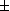# Graphic addition --- Introduction ---

Graphic addition is an exercise concerning the graphical recognition of the addition of functions of one real variable.

The server will give you the graph of two functions f (x) and g(x), whose expressions will be hidden. You will then be presented a certain number of other graphs, corresponding to functions such as f (x)+g(x), f (-x)-g(x), -f (x)+g(-x), etc (see the menu below). And you should locate the one which corresponds to one which will be precised in a question. To give the reply, you can simply click on the graph which you believe to be the good one.

The exercise can be configured by the following parameters.
• Type of the function to recognize:
1.f (x)g(x) ;
2. f (x)+g(x) ;
3.f (x)g(x) ;
4. f (x)g(x) ;
5.f (x)0.5g(x),0.5f (x)g(x) ;
• Numbre of questions in one session: , , , , , , , (The score is attributed only at the end of a session.)
• or you can be asked to recognize each presented curve.

Other exercises on: graphic   Functions   Addition

In order to access WIMS services, you need a browser supporting forms. In order to test the browser you are using, please type the word wims here: and press Enter''.